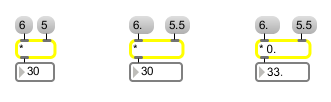# *

Multiply two numbers, output the result

## Description

* takes any two given numbers, multiplies them together, and outputs the result upon receiving input in the left inlet.

## Arguments

Name Type Opt Description
initial-multiplier int or float opt Sets the initial value, to be multiplied by a number received in the left inlet. A float argument causes the numbers to be multiplied as floats.

## Messages

 bang In left inlet: Performs the multiplication with the numbers currently stored. If there is no argument, * initially holds 0 as a multiplier. int input [int] In left inlet: The number is multiplied by the number in the right inlet, and the result is sent out the outlet. (inlet1) multiplier [int] In right inlet: The number is stored for multiplication with a number received in the left inlet. float input [float] Converted to int before multiplication, unless * has a float argument. set set-input [int] In left inlet: The word set, followed by a number, sets the inlet's input without causing output. list input [list] In left inlet: The first number is multiplied by the second number, and the result is sent out the outlet.

## Output

float: Only if there is an argument with a decimal point.
int: The product of the two numbers received in the inlets.

## ExamplesMultiplied as ints... Floats are truncated before multiplication... ...unless there is a float argument